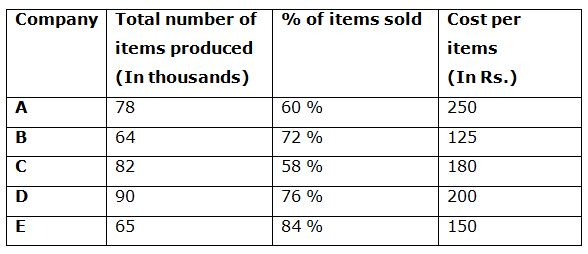# CWC/FCI Prelims 2019 – Quantitative Aptitude Questions (Day-55)

Dear Readers, Exam Race for the Year 2019 has already started, CWC/ FCI will be the most expected exam among the aspirants. Preparation and performance both requisite to clear CWC/FCI Exam. To enrich your preparation here we are providing a new series of Practice Questions on CWC/ FCI Quantitative Aptitude Section. Aspirants, practice these questions on a regular basis to improve your score in the aptitude section. Start your effective preparation from the right beginning to get success in the upcoming CWC/FCI Exam.

[WpProQuiz 6176]

Directions (1 – 5): What value should come in place of (?) in the following questions?

1) 15 (5/14) + 12 (3/7) – 5 (½) – 7 (1/7) =? + 4 (3/14)

a) 10 (13/14)

b) 9 (11/14)

c) 11 (6/7)

d) 9 (3/7)

e) None of these

2) 6800 ÷ 8 + (3/7) of 8750 + 18 % of 1500 =?2 – 755

a) 70

b) 65

c) 60

d) 75

e) None of these

3) √4225 + 5832 + 18 % of 1500 =? – 47

a) 280

b) 400

c) 360

d) 420

e) None of these

4) (3/8) of 2432 of 275 ÷ 25 + 576 ÷ 12 =?

a) 9260

b) 12040

c) 10080

d) 8520

e) None of these

5) 24 % of 1600 + 18 % of 2400 – 255 ÷ 5 =?

a) 765

b) 650

c) 825

d) 930

e) None of these

Directions (6 – 10): Study the following information carefully and answer the given questions.

The following table shows the total number items produced by 5 different companies (In thousands) and the percentage of items sold and the cost per item.6) Total number of items sold by the company A is approximately what percentage of total number of items sold by the company C?

a) 98 %

b) 90 %

c) 80 %

d) 115 %

e) 65 %

7) Find the total cost earned by the company B and D together?

a) 1.728 Crore

b) 1.856 Crore

c) 1.642 Crore

d) 1.944 Crore

e) None of these

8) Find the ratio between the total number of items remains unsold by the company B to that of company D?

a) 125: 136

b) 112: 135

c) 57: 77

d) 48: 69

e) None of these

9) Find the difference between the total cost earned by the company A to that of company E?

a) 0.351 Crore

b) 3.51 Crore

c) 0.0351 Crore

d) 35.1 lakhs

e) None of these

10) Total number of items produced by the company A and D together is approximately what percentage more/less than the total number of items produced by the company B and C together?

a) 25 % more

b) 20 % less

c) 15 % more

d) 15 % less

e) 20 % more

15 (5/14) + 12 (3/7) – 5 (½) – 7 (1/7) – 4 (3/14) = x

X = (15 + 12 – 5 – 7 – 4) (5/14 + 3/7 – ½ – 1/7 – 3/14)

X = 11 [(5 + 6 – 7 – 2 – 3)/14]

X = 11 (-1/14)

X = (154 – 1)/14 = 153/14 = 10 (13/14)

6800 ÷ 8 + (3/7) of 8750 + 18 % of 1500 = x2 – 755

(6800/8) + (3/7)*8750 + (18/100)*1500 + 755 = x2

x2 = 850 + 3750 + 270 + 755 = 5625

x = 75

√4225 + ∛5832 + 18 % of 1500 = x – 47

65 + 18 + (18/100)*1500 + 47 = x

x = 65 + 18 + 270 + 47 = 400

(3/8)*2432*(275/25) + (576/12) = x

X = 10032 + 48 = 10080

(24/100)*1600 + (18/100)*2400 – (255/5) = x

384 + 432 – 51 = x

x = 765

Directions (6-10):

Total number of items sold by the company A

= > 78*(60/100)

Total number of items sold by the company C

= > 82*(58/100)

Required % = {[78*(60/100)]/ [82*(58/100)]}*100 = 98 %

The total cost earned by the company B and D together

= > 64*(72/100)*125 + 90*(76/100)*200

= > (5760 + 13680) thousands

= > 1.944 Crore

The total number of items remains unsold by the company B

= > 64*(28/100)

The total number of items remains unsold by the company D

= > 90*(24/100)

Required ratio = [64*(28/100)]: [90*(24/100)] = 112: 135

The total cost earned by the company A

= > 78*(60/100)*250 = 11700 thousand

= > 1.17 Crore

The total cost earned by the company E

= > 65*(84/100)*150 = 8190 thousand

= > 0.819 Crore

Required difference = 1.17 – 0.819 = 0.351 Crore

Total number of items produced by the company A and D together

= > 78 + 90 = 168000

Total number of items produced by the company B and C together

= > 64 + 82 = 146000

Required % = [(168000 – 146000)/146000]*100 = 15 % more

Related Articles: